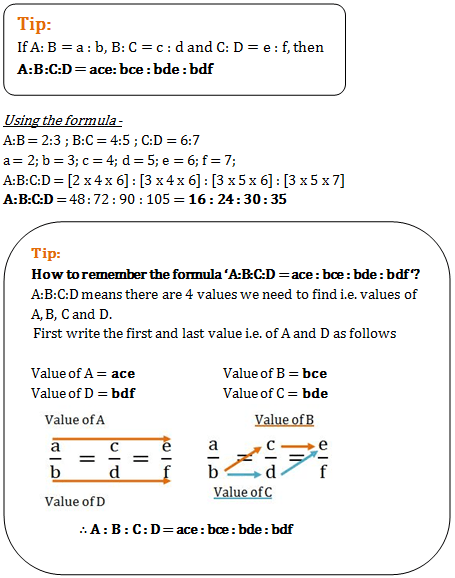# Ratio and Proportion - Quantitative Aptitude (MCQ) questions for Q. 29211

Q.  If A:B = 2:3, B:C = 4:5 and C:D = 6:7, then A:B:C:D is
- Published on 07 Jul 17

a. 18:24:30:35
b. 16:24:30:35
c. 16:22:30:35
d. 16:24:15:35#### Discussion

• B.Veeresh   -Posted on 07 Aug 18
Simple method..2:3:3:3
4:4:5:5
6:6:6:7
2*4*6=48..3*4*6=72..3*5*6=90..3*5*7=105..there are divisible is 3